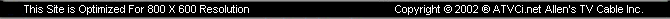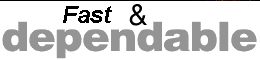Click on a link below!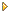Speed LevelsAdvancedRequirementsSupportDownloadsConfigurationsWebMailInternet LinksContact UsLocationsAbout UsHome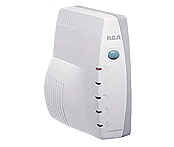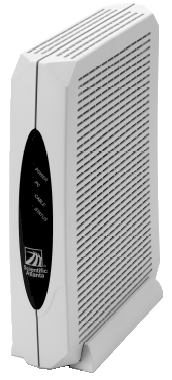ATVCi.net  Use'sDOCSIS Modems
Look for this LogoBits & Bytes Reference
2001

Abbreviations like Kb, KB, Mb and GB are often confused, misunderstood or used incorrectly. Here's a brief explanation of proper usage.

Let's start at the beginning. The only data that a computer can understand is on and off. But those two simple commands can be grouped into millions of combinations and it is the way they are grouped in series that creates complex data.

The basic unit is called a bit (binary digit). Each bit has an electronic switch, or gate. If the gate is open the bit is on and electricity can go through. The computer reads on or open switches as a number 1. If the gate is closed or off, the electricity is blocked and the computer reads off bits as 0.

Bits can be grouped together to allow more complex code. Eight bits are grouped together to form a byte. This allows 256 possible combinations of 1/0. The abbreviation for bit is a lowercase "b"; the abbreviation for byte is an uppercase "B". Bits and bytes can then be expressed in larger measurements. They are generally expressed in exponents of two, known as binary code.

The Standards

Although computer data is normally measured in binary code, the prefixes for the multiples are based on the metric system. The nearest binary number to 1,000 is 2^10 or 1,024; thus 1,024 bytes was named a Kilobyte. So, although a metric "kilo" equals 1,000 (e.g. one kilogram = 1,000 grams), a binary "Kilo" equals 1,024 (e.g. one Kilobyte = 1,024 bytes). Not surprisingly, this has led to a great deal of confusion.

In December 1998 the International Electrotechnical Commission (IEC) approved a new IEC International Standard. Instead of using the metric prefixes for multiples in binary code, the new IEC standard invented specific prefixes for binary multiples made up of only the first two letters of the metric prefixes and adding the first two letters of the word "binary". Thus, for instance, instead of Kilobyte (KB) or Gigabyte (GB), the new terms would be kibibyte (KiB) or gibibyte (GiB).

Here are brief summaries of the Old Standard and the IEC Standard:

 Old Standard bit b 0 or 1 byte B 8 bits kilobit kb 1000 bits Kilobyte (binary) KB 1024 bytes Kilobyte (decimal) KB 1000 bytes Megabit Mb 1000 kilobits Megabyte (binary) MB 1024 Kilobytes Megabyte (decimal) MB 1000 Kilobytes Gigabit Gb 1000 Megabits Gigabyte (binary) GB 1024 Megabytes Gigabyte (decimal) GB 1000 Megabytes

 IEC Standard bit bit 0 or 1 byte B 8 bits kibibit Kibit 1024 bits kilobit kbit 1000 bits kibibyte (binary) KiB 1024 bytes kilobyte (decimal) kB 1000 bytes megabit Mbit 1000 kilobits mebibyte (binary) MiB 1024 kibibytes megabyte (decimal) MB 1000 kilobytes gigabit Gbit 1000 megabits gibibyte (binary) GiB 1024 mebibytes gigabyte (decimal) GB 1000 megabytes

____________________________________________________

Binary Code

When used to describe Memory Size, or Data Storage, bits/bytes are generally calculated as some exponent of two:

 Old Standard 1 bit (b) 1 byte (B) = 8 bits 1 Kilobyte (K / KB) = 2^10 bytes = 1,024 bytes 1 Megabyte (M / MB) = 2^20 bytes = 1,048,576 bytes 1 Gigabyte (G / GB) = 2^30 bytes = 1,073,741,824 bytes 1 Terabyte (T / TB) = 2^40 bytes = 1,099,511,627,776 bytes Note: In the metric system the "k" or "kilo" prefix is always lowercase but since these binary uses are not properly metric, it has become standard to use an uppercase "K" for the binary form.

 IEC Standard 1 bit (bit) 1 byte (B) = 8 bits 1 kibibyte (KiB) = 2^10 bytes = 1,024 bytes 1 mebibyte (MiB) = 2^20 bytes = 1,048,576 bytes 1 gibibyte (GiB) = 2^30 bytes = 1,073,741,824 bytes 1 tebibyte (TiB) = 2^40 bytes = 1,099,511,627,776 bytes Note: For consistency with the other prefixes for binary multiples, the initial "K" used in the abbreviation for kibibyte (2^10) has been capitalised.

Decimal System

Although data storage capacity is generally expressed in binary code, many hard drive manufacturers (and some newer BIOSs) use a decimal system to express capacity:

 Old Standard 1 bit (b) 1 byte (B) = 8 bits 1 Kilobyte (K / KB) = 10^3 bytes = 1,000 bytes 1 Megabyte (M / MB) = 10^6 bytes = 1,000,000 bytes 1 Gigabyte (G / GB) = 10^9 bytes = 1,000,000,000 bytes 1 Terabyte (T / TB) = 10^12 bytes = 1,000,000,000,000 bytes Note: A third definition of Megabyte is that used in formatting floppy disks: 1 Megabyte = 1,024,000 bytes.

 IEC Standard 1 bit (bit) 1 byte (B) = 8 bits 1 kilobyte (kB) = 10^3 bytes = 1,000 bytes 1 megabyte (MB) = 10^6 bytes = 1,000,000 bytes 1 gigabyte (GB) = 10^9 bytes = 1,000,000,000 bytes 1 terabyte (TB) = 10^12 bytes = 1,000,000,000,000 bytes Note: Note the use of a lowercase "k" in the abbreviation for kilobyte, in keeping with the metric system.

_______________________________________________

Metric Code

When used to describe Data Transfer Rate, bits/bytes are calculated as in the metric system:

 Old Standard 1 bit (b) = 0 or 1 = one binary digit 1 kilobit (kb / Kb / K) = 10^3 bits = 1,000 bits 1 Megabit (Mb) = 10^6 bits = 1,000,000 bits 1 Gigabit (Gb) = 10^9 bits = 1,000,000,000 bits Note: Kilobits per second is usually shortened to kbps or Kbps. Although technically speaking, the term kilobit should have a lowercase initial letter, it has become common to capitalise it in abbreviation (e.g. "56 Kbps" or "56K"). The simple "K" might seem ambiguous but, in the context of data transfer, it can be assumed that the measurement is in bits rather than bytes unless indicated otherwise.

 IEC Standard 1 bit (bit) = 0 or 1 = one binary digit 1 kilobit (kbit) = 10^3 bits = 1,000 bits 1 megabit (Mbit) = 10^6 bits = 1,000,000 bits 1 gigabit (Gbit) = 10^9 bits = 1,000,000,000 bits

_______________________________________________